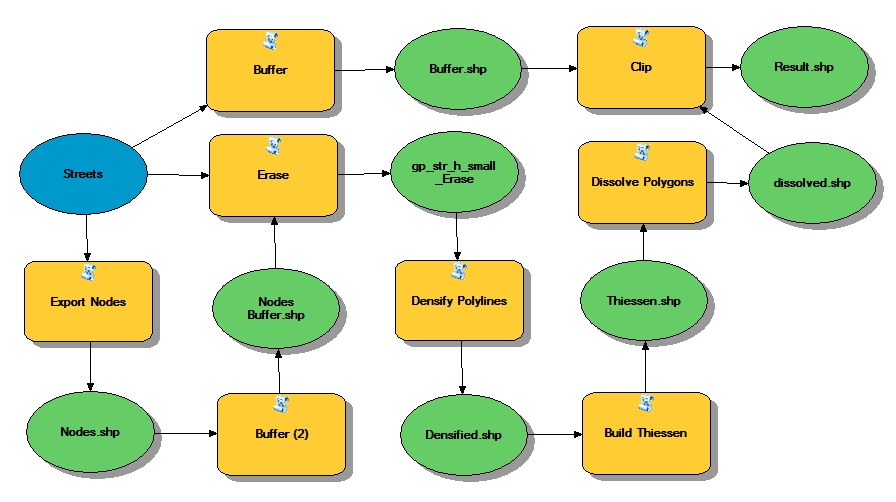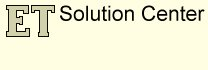## Creating non-ovelapping buffers with attributes for Polylines

In this article we presented an approach for creating non-overlapping buffers for points (one polygon per original point) that will carry the attributes of the original points. The procedure will work if the input dataset represents points. Here we are trying to propose a method to achieve the same goal if the input features are polylines.

Only functions available in ET GeoWizards 12.0 are used.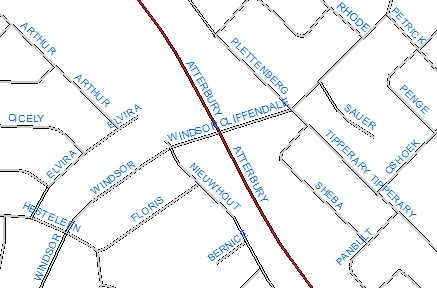Source dataset - We have a topologically correct (node at each intersection) street dataset that we want to buffer.  We want however the buffer polygons to carry the Street names and some other attributes available in the input.The Buffer function (if no Dissolve option is used) will give us a polygon per original feature. Each polygon will carry the attributes of the original feature. The problem however is that the buffer polygons will overlap.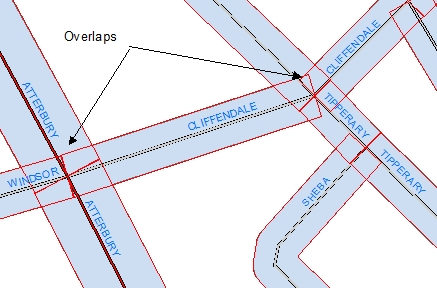We'll get a similar result with the Buffer Polylines function (if no Dissolve option is used) with the "Flat" end option.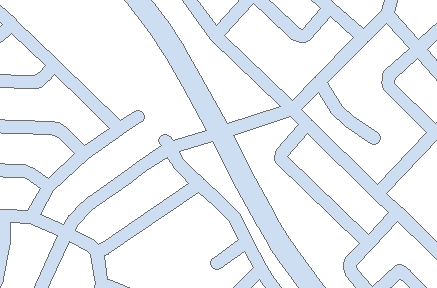STEP 1: If we use the Buffer function (with Dissolve option but no dissolve attributes specified), we will get non overlapping buffers, but the original attributes cannot be attached to the buffers because there will be many to one relationship (many streets will be represented by a single polygon).
After having created the buffer, there are two major problems that we need to solve:

1. We need to partition the global buffer polygon in a way that each street segment is represented by a single polygon.
2. We need to attach the attributes from the original streets to the individual polygons polygons.The partitioning the space can be solved in the same way as for the points. If however the source dataset is polyline there are a couple of complications:
The first problem is that if the street dataset is topologically correct, then in the intersections each polyline that participates will have a node. This means that there will be several (depending on how many street segments connect) data points at each intersection. The best way to solve the problem is to eliminate these data points.
We can achieve this in a 3 step process:

STEP 2: Use the Export Nodes function to get the regular nodes (where 3 or more streets intersect) of the street dataset.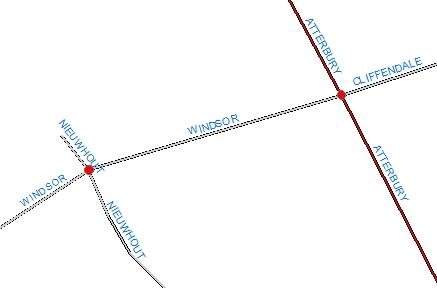STEP 3: Buffer the nodes with a very small buffer distance (at least smaller than the buffer distance used)STEP 4: Use the Erase function to remove the parts of the polylines that are inside the buffer created above.

The second problem is that in some case the distance between the adjacent vertices in a polyline might be much longer than the buffer distance, we need to introduce some new vertices.

STEP 5: Use the Densify function with a tolerance smaller or equal to the buffer distance. If different buffer distances are used for the different polylines, the smallest buffer distance must be used.STEP 6: Use the Build Thiessen Polygons function to partition the space. Use the Attach attribute option because we'll need the attributes of the original polylines.
The function will create a polygon around each vertex.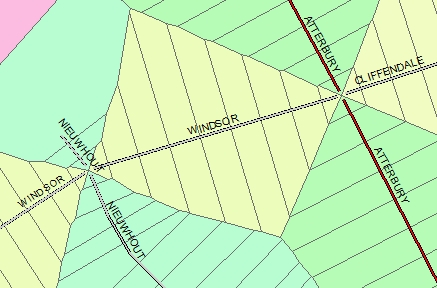As can be seen from the detailed picture on the left, the space in the proximity of the nodes is partitioned as desired.STEP 7: Now we need just the parts of the Thiessen polygons that overlaps the Buffer created in STEP 1. We can use the Clip function to get this.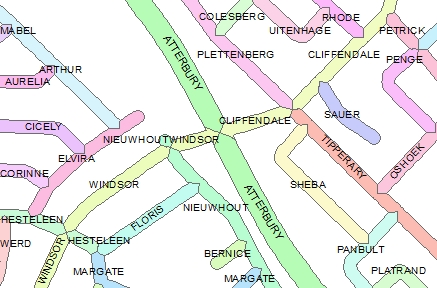STEP 8: Use the Dissolve Polygons to merge the Thiessen polygons pertaining to a single street together.Detail of the resulting polygons having the attributes of the original street polylines

#### Sample Model of the entire process (ArcGIS Desktop and ArcGIS Pro only)